Courses

# Notes | EduRev

## Class 10 : Notes | EduRev

The document Notes | EduRev is a part of the Class 10 Course Mathematics (Maths) Class 10.
All you need of Class 10 at this link: Class 10

NCERT TEXTBOOK QUESTIONS SOLVED
Page No. 257
EXERCISE 13.4

[Use π = 22/7, unless stated otherwise]
Q 1. A drinking glass is in the shape of a frustum of a cone of height 14 cm. The diameters of its two circular ends are 4 cm and 2 cm. Find the capacity of the glass.
Sol. Given, upper diameter - 4 cm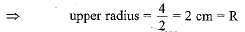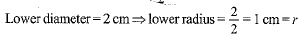Height of glass = 14 cm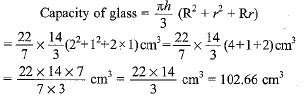Q 2. The slant height of a frustum of a cone is 4 cm and the perimeters (circumference) of its circular ends are 18 cm and 6 cm. Find the curved surface area of the frustum.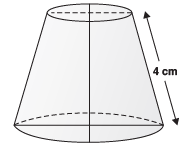Sol. We have:
Slant height (l) = 4 cm
2πr1 = 18 cm
and 2πr2 = 6 cm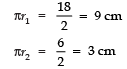∴ Curved surface area of the frustum of the cone
= π (r1 + r2) l = (πr1 + πr2) l = (9 + 3) × 4 cm2 = 12 × 4 cm2 = 48 cm2.

Q 3. A fez, the cap used by the Turks, is shaped like the frustum of a cone (see Fig.). If its radius on the open side is 10 cm, radius at the upper base is 4 cm and its slant height is 15 cm, find the area of material used for making it.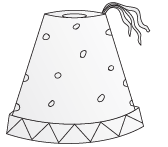Sol. Here, the radius of the open side (r1) = 10 cm
The radius of the upper base (r2) = 4 cm
Slant height (l) = 15 cm
∴ Area of the material required
= [Curved surface area of the frustum] + [Area of the top end]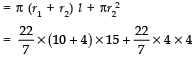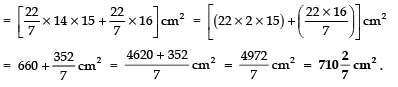Q 4. A container, opened from the top and made up of a metal sheet, is in the form of a frustum of a cone of height 16 cm with radii of its lower and upper ends as 8 cm and 20 cm, respectively. Find the cost of the milk which can completely fill the container, at the rate of Rs 20 per litre. Also find the cost of metal sheet used to make the container, if it costs Rs 8 per 100 cm2. (Take π = 3.14)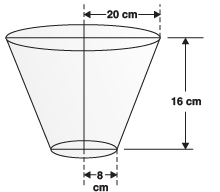Sol. We have:
r1 = 20 cm, r2 = 8 cm
and h = 16 cm
∴ Volume of the frustum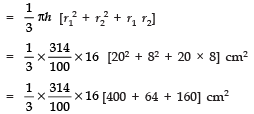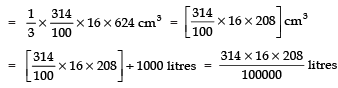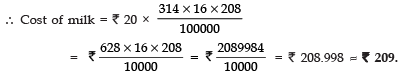Now, slant height of the given frustum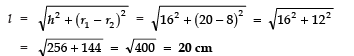∴ Curved surface area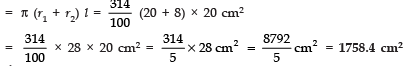Area of the bottom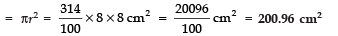∴ Total area of metal required = 1758.4 cm2 + 200.96 cm2 = 1959.36 cm2
Cost of metal required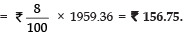Q 5. A metallic right circular cone 20 cm high and whose vertical angle is 60° is cut into two parts at the middle of its height by a plane parallel to its base. If the frustum so obtained be drawn into a wire of diameter 1/16 cm , find the length of the wire.
Sol. Let us consider the frustum DECB of the metallic cone ABC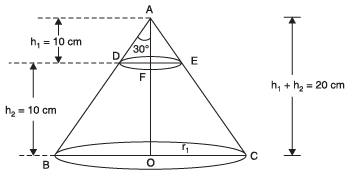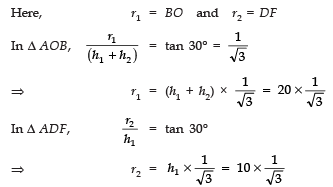Now, the volume of the frustum DBCE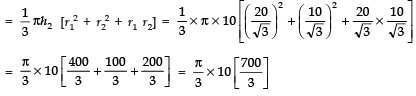Let l be the length and D be diameter of the wire drawn from the frustum. Since the wire is in the form of a cylinder,
∴ Volume of the wire = πr2l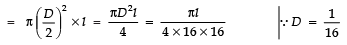∵ [Volume of the frustum] = [Volume of the wire]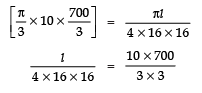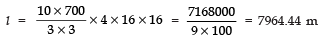Thus, the required length of the wire = 7964.44 m.

Offer running on EduRev: Apply code STAYHOME200 to get INR 200 off on our premium plan EduRev Infinity!

,

,

,

,

,

,

,

,

,

,

,

,

,

,

,

,

,

,

,

,

,

;